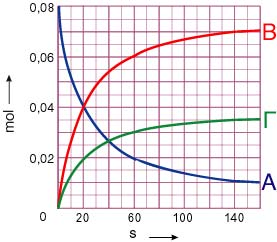1. Solution
a) υ = k[A]    ν = 1 first order reaction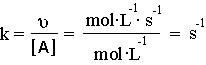b) υ = k[A][B]    ν =1+1=2 second order reaction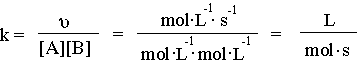c) υ = k[A][B]2    ν = 1+2=3 third order reaction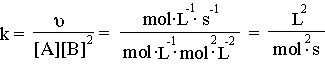2.Solution
Due to the fact that the reaction is simple, the rate is given by
υ = k[A][B]2    (1)
a) If we double the concentration of A the rate becomes
υ1 = k[2A][B]2 = 2k[A][B]2    (2)
From (1) and (2) we have υ1 = 2υ. Therefore the rate of the reaction is doubled.
b)
If we double the concentration of B the rate becomes
υ2 = k[A][2B]2 = 4k[A][B]2    (3)
From (1) and (3) we have υ1 = 4υ. Therefore the rate of the reaction is quadrupled.
c) If we dilute the solution until it becomes twice its original volume, the concentrations decrease to one half their initial value. The rate low gives us:
υ3 = k[0.5A][0.5B]
2 = 0.125k[A][B]2     (4)

From (1) and (4) we get   υ3 = 0.125υ = υ/8.  Therefore the rate of the reaction decreases to one eighth its initial value.

3. Solution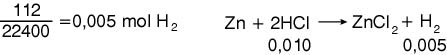From the reaction we can see that 0.001 moles of HCl are consumed.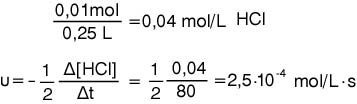a) 0.025 mol/L·s,   0.025 mol/L·s    b)zero.

α) 0.025 mol/L·s  and  0.011 mol/L·s
β)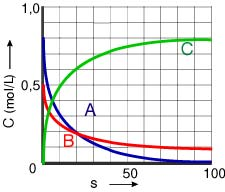Return to dictation

Either 2 or 8.

7 Solution
The analogy 1:2 means that, if the quantity of A is a moles, B would be 2a moles.
The evolution of the reaction is described as follows: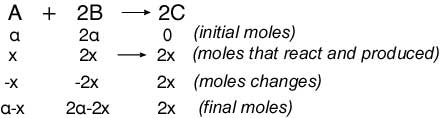From the stoichiometry of the reaction and the initial quantities,results that the reactants are fully consumed. Thus,
α - x = 0 =>α = x. From this result we conclude that ,after the termination of the reaction the quantities of A and B are fully consumed. So, the curves are diminishing and have a tendancy to the zero axis 0 (t).
Therefore, the demanded diagram is: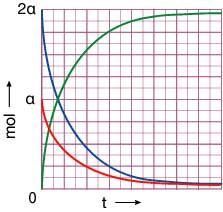Return to dictation

α)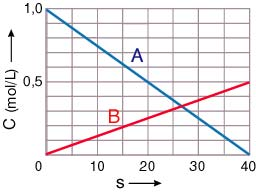β) 0.0125 mol/L.s

a 2,  b 6,  c 3,  d 5.

α) 0.02 mol/L·s, 0.01 mol/L·s, 0.005 mol/L·s, 0.0025 mol/L·s, 0.009375 mol/L·s,
β)11. Solution
The progress of the reaction is described as follows: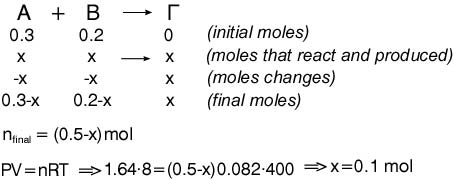a) 0.2 mol, 0.1 mol and 0.1 mol

β)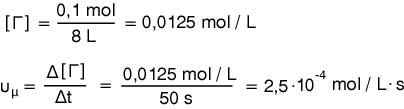12. Solution
The rate is given from the type: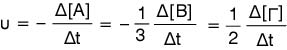So, the demanded rates of consumption and production are: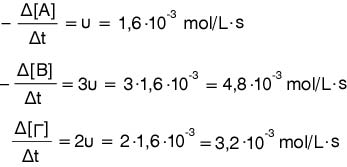υ = -Δ[Α] / Δt => -Δ[Α] = υ·Δt = 1,6·10-3·50 = 0,08 mol/L So, the total quantity of A that is consumed is 2,5.0,08 = 0,2 mol.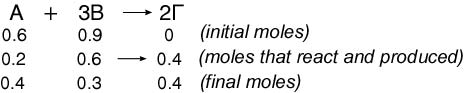Therefore, the composition of the mixture after the elapse of the 50 seconds is:
0,4 mol  Α,  0.3 mol  Β and 0.4  Γ.

13. Solution

Due to the fact that we don’t know whether the reaction is simple (one step only) or not and we don’t have any other relevant information, we ignore the way it was inscribed earlier and we write the rate low as follows:

υ = k[A]x[B]y
From this type we get the following equations for each one of the measurements.

4·10-3 = k 0.1x0.1  (1)

8·10-3 = k 0.2x0.1y    (2)

1.6·10-2 = k 0.1x0.1y   (3)

From (1) and (2) we get: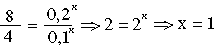From (1) and (3) we get: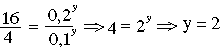a) The rate low is given from the type υ = k[A][B] 2

b) The reaction’s order is ν = 1+2 = 3 (Third order)

c) From (1) we get: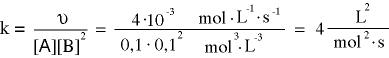b)  ν = 3,    c) 0.5L3 mol-3·s-1,    d) 7.5·10-3 mol·L-1·sec

b) ν=4,       c) k=9L3·mol-3·s-1

16. Solution

We know that the relative pressure of a gas, component of a mixture, is given by the equation P=[A]RT, which gives us [A]=Pa/RT. From this relationship we can see that the relative pressure of a gas constitutes a way to measure the gas’s concentration in the gas mixture. According to the above, the arithmetical values of the relative pressures of the reactants, are equal to the relative values of their concentrations. Therefore the rate low, for the above reaction, it can also be written in the following form: υ = k·PAx PBy  where k is a constant with the suitable units (dimensions).
As we did in the solution of exercise 3, in order to write down the rate low we ignore the reaction’s coefficients, because we don’t know from the dictation whether the reaction is simple (one step only) or not. According to the above expression of the rate low, we get for each one of the measurements:

1.25·10-3 = k 0.2x0.25y (1)

2.5·10-3 = k 0.4x0.125y (2)

5·10-3 = k 0.4x 0.25y (3)

from (1) and (3) we get: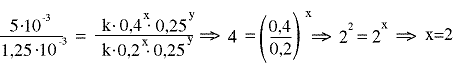from (2) and (3) we get: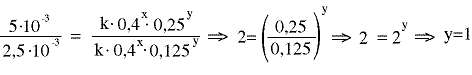The rate low is given by: υ = k·PA2PB

The reaction is third order because ν = 2+1= 3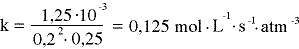ν = 2,   k = 5·10-3 mol·L-1 s-1 atm-2

a) ν = 0,    b) mol/L·sec

19. Solution
The initial concentrations are: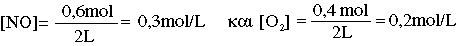The progress of the reaction appears in the following table
2ΝΟ    +      O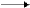2ΝΟ2
0.3             0.2           0     (initial mol/L)
0.2             0.10.2   (mol/L that react and produced)
0.1             0.1          0.2   (final mol/L)
From dictation we know that the reaction is simple (only one step), which dives us:

υ = k[NO]2[O2].
This type for the initial situation becomes: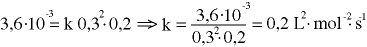For the final situation we have:

υ2 = 0.2·0.12 0.1 = 2·10-4 mole·L-1s-1

0.45 ,    0.45   and  0.9 mol

21. Solution

α) 6.4 / 80 = 0.08 mol of Α.

The progress of the reaction is described as follows: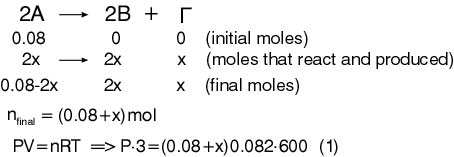From equation (1) for P=1.64 atm we get 0.02 moles. So, at the specific time we have 0.04 moles of A, 0.04 moles of B and 0.02 moles of C. The rate is given by the type:
υ
1 = k(0.04/3)2  (2)
Also, from equation (1) for P=1.804 atm we get 0.03 moles. Therefore at the specific time we have 0.02 moles of A, 0.06 moles of B and 0.03 moles of C. the rate is given by the type:
υ
2 = k(0.02/3)2  (3)
From (2) and (3) we get:  υ
12 = 4:1
β)